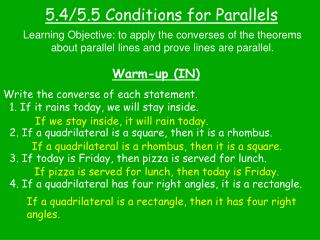DownloadDownload Presentation5.4/5.5 Conditions for Parallels

# 5.4/5.5 Conditions for Parallels

Télécharger la présentation## 5.4/5.5 Conditions for Parallels

- - - - - - - - - - - - - - - - - - - - - - - - - - - E N D - - - - - - - - - - - - - - - - - - - - - - - - - - -
##### Presentation Transcript

1. 5.4/5.5 Conditions for Parallels Learning Objective: to apply the converses of the theorems about parallel lines and prove lines are parallel. Warm-up (IN) Write the converse of each statement. 1. If it rains today, we will stay inside. 2. If a quadrilateral is a square, then it is a rhombus. 3. If today is Friday, then pizza is served for lunch. 4. If a quadrilateral has four right angles, it is a rectangle. If we stay inside, it will rain today. If a quadrilateral is a rhombus, then it is a square. If pizza is served for lunch, then today is Friday. If a quadrilateral is a rectangle, then it has four right angles.

2. Learning Objective: to apply the converses of the theorems about parallel lines and prove lines are parallel. Notes **Converses of the Parallel lines theorems Corresponding angles - lines Alternate interior angles - lines Same-side interior angles - lines

3. Learning Objective: to apply the converses of the theorems about parallel lines and prove lines are parallel, and to construct parallel lines. X Y (4x+15) (9x-25) Z W B Ex 1 – Find the value of x that will allow you to prove that WXYZ is a trapezoid. lines 4x+15 9x-25 = 40 5x = x = 8 Ex 2 – Prove that if 2 sides of a quad. are parallel, and one pair of opposite angles are congruent, then the quad. is a parallelogram. Given: OR//FT R O FORT is a parallelogram Prove: F T

4. Given: OR//FT R O FORT is a parallelogram Prove: F T Reasons Statements Given 1. 1. OR//FT 2. Def cong. 3. 4. Subst. 5. OF//RT 5. lines 6. FORT is a parallelogram 6. Def parallelogram

5. n m t **2 more ways to prove lines parallel! Dual Perpendiculars Theorem - In a plane, if 2 lines are both perpendicular to a 3rd line, then the 2 lines are parallel. Dual Parallels Theorem - If 2 lines are both parallel to a 3rd line, then the 2 lines are parallel. m n t

6. Out – What do you use the converses of the parallel lines theorems for? Summary – I might still need help on…. Quiz tomorrow!!! HW – p. 245 #1-7, 16 p. 253 #1-3, 7-10,23